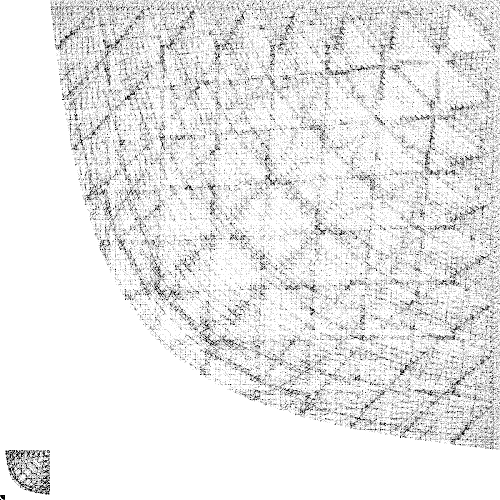Vampire Numbers Visualized

Vampire numbers are fairly interesting. Vampire numbers are numbers whose product contains the same digits as the two vampire numbers. For example, 21 x 60 = 1260. The two vampire numbers (called “fangs”) must be the same length and both cannot end with a zero. A longer example is 68088 x 45321 = 3085816248. You can find out more about these numbers on Wikipedia.

It’s fairly simple to write a program to calculate vampire numbers. I was somewhat bored and went ahead and wrote one up. Download the vampire number generator.

I’ve gone ahead and calculated all of the vampire numbers up to five digits. You can download the full text file of vampire numbers here (zipped, it’s about 1.06 MB). The nifty thing is, if you plot these numbers on an image with the two fangs being the X and Y coordinates, you get a pretty interesting image. This image is 500×500 pixels and covers the range of 0 to 100,000. Each pixel represents a 20×20 range of numbers. The more dense this area is with fangs, the darker the pixel is colored in. The 0, 0 origin is in the lower left. The final image has an interesting pattern: